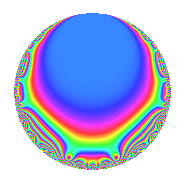# Properties

 Label 5610.2.a.byLevel 5610 Weight 2 Character orbit 5610.a Self dual Yes Analytic conductor 44.796 Analytic rank 0 Dimension 3 CM No Inner twists 1

# Related objects

## Newspace parameters

 Level: $$N$$ = $$5610 = 2 \cdot 3 \cdot 5 \cdot 11 \cdot 17$$ Weight: $$k$$ = $$2$$ Character orbit: $$[\chi]$$ = 5610.a (trivial)

## Newform invariants

 Self dual: Yes Analytic conductor: $$44.7960755339$$ Analytic rank: $$0$$ Dimension: $$3$$ Coefficient field: $$\Q(\zeta_{18})^+$$ Coefficient ring: $$\Z[a_1, \ldots, a_{7}]$$ Coefficient ring index: $$2^{3}$$ Fricke sign: $$-1$$ Sato-Tate group: $\mathrm{SU}(2)$

## $q$-expansion

Coefficients of the $$q$$-expansion are expressed in terms of a root $$\beta$$ of the polynomial $$x^{3} - 3 x - 1$$. We also show the integral $$q$$-expansion of the trace form.

 $$f(q)$$ $$=$$ $$q - q^{2} + q^{3} + q^{4} - q^{5} - q^{6} + 2 \beta q^{7} - q^{8} + q^{9} +O(q^{10})$$ $$q - q^{2} + q^{3} + q^{4} - q^{5} - q^{6} + 2 \beta q^{7} - q^{8} + q^{9} + q^{10} + q^{11} + q^{12} + ( -6 - 2 \beta + 4 \beta^{2} ) q^{13} -2 \beta q^{14} - q^{15} + q^{16} + q^{17} - q^{18} + ( -6 - 2 \beta + 4 \beta^{2} ) q^{19} - q^{20} + 2 \beta q^{21} - q^{22} + ( -2 + 2 \beta ) q^{23} - q^{24} + q^{25} + ( 6 + 2 \beta - 4 \beta^{2} ) q^{26} + q^{27} + 2 \beta q^{28} + ( 2 + 4 \beta ) q^{29} + q^{30} + ( 8 + 4 \beta - 4 \beta^{2} ) q^{31} - q^{32} + q^{33} - q^{34} -2 \beta q^{35} + q^{36} + 2 q^{37} + ( 6 + 2 \beta - 4 \beta^{2} ) q^{38} + ( -6 - 2 \beta + 4 \beta^{2} ) q^{39} + q^{40} + ( -12 - 4 \beta + 4 \beta^{2} ) q^{41} -2 \beta q^{42} + ( 4 - 2 \beta ) q^{43} + q^{44} - q^{45} + ( 2 - 2 \beta ) q^{46} + q^{48} + ( -7 + 4 \beta^{2} ) q^{49} - q^{50} + q^{51} + ( -6 - 2 \beta + 4 \beta^{2} ) q^{52} + ( 12 - 2 \beta - 4 \beta^{2} ) q^{53} - q^{54} - q^{55} -2 \beta q^{56} + ( -6 - 2 \beta + 4 \beta^{2} ) q^{57} + ( -2 - 4 \beta ) q^{58} + ( -4 - 2 \beta ) q^{59} - q^{60} + ( 6 - 4 \beta^{2} ) q^{61} + ( -8 - 4 \beta + 4 \beta^{2} ) q^{62} + 2 \beta q^{63} + q^{64} + ( 6 + 2 \beta - 4 \beta^{2} ) q^{65} - q^{66} + ( 6 - 4 \beta ) q^{67} + q^{68} + ( -2 + 2 \beta ) q^{69} + 2 \beta q^{70} + ( 4 + 2 \beta ) q^{71} - q^{72} + ( 18 + 10 \beta - 8 \beta^{2} ) q^{73} -2 q^{74} + q^{75} + ( -6 - 2 \beta + 4 \beta^{2} ) q^{76} + 2 \beta q^{77} + ( 6 + 2 \beta - 4 \beta^{2} ) q^{78} + ( -6 - 2 \beta + 4 \beta^{2} ) q^{79} - q^{80} + q^{81} + ( 12 + 4 \beta - 4 \beta^{2} ) q^{82} + 2 \beta q^{84} - q^{85} + ( -4 + 2 \beta ) q^{86} + ( 2 + 4 \beta ) q^{87} - q^{88} + ( 14 - 8 \beta^{2} ) q^{89} + q^{90} + ( 8 + 12 \beta - 4 \beta^{2} ) q^{91} + ( -2 + 2 \beta ) q^{92} + ( 8 + 4 \beta - 4 \beta^{2} ) q^{93} + ( 6 + 2 \beta - 4 \beta^{2} ) q^{95} - q^{96} + ( 4 - 8 \beta ) q^{97} + ( 7 - 4 \beta^{2} ) q^{98} + q^{99} +O(q^{100})$$ $$\operatorname{Tr}(f)(q)$$ $$=$$ $$3q - 3q^{2} + 3q^{3} + 3q^{4} - 3q^{5} - 3q^{6} - 3q^{8} + 3q^{9} + O(q^{10})$$ $$3q - 3q^{2} + 3q^{3} + 3q^{4} - 3q^{5} - 3q^{6} - 3q^{8} + 3q^{9} + 3q^{10} + 3q^{11} + 3q^{12} + 6q^{13} - 3q^{15} + 3q^{16} + 3q^{17} - 3q^{18} + 6q^{19} - 3q^{20} - 3q^{22} - 6q^{23} - 3q^{24} + 3q^{25} - 6q^{26} + 3q^{27} + 6q^{29} + 3q^{30} - 3q^{32} + 3q^{33} - 3q^{34} + 3q^{36} + 6q^{37} - 6q^{38} + 6q^{39} + 3q^{40} - 12q^{41} + 12q^{43} + 3q^{44} - 3q^{45} + 6q^{46} + 3q^{48} + 3q^{49} - 3q^{50} + 3q^{51} + 6q^{52} + 12q^{53} - 3q^{54} - 3q^{55} + 6q^{57} - 6q^{58} - 12q^{59} - 3q^{60} - 6q^{61} + 3q^{64} - 6q^{65} - 3q^{66} + 18q^{67} + 3q^{68} - 6q^{69} + 12q^{71} - 3q^{72} + 6q^{73} - 6q^{74} + 3q^{75} + 6q^{76} - 6q^{78} + 6q^{79} - 3q^{80} + 3q^{81} + 12q^{82} - 3q^{85} - 12q^{86} + 6q^{87} - 3q^{88} - 6q^{89} + 3q^{90} - 6q^{92} - 6q^{95} - 3q^{96} + 12q^{97} - 3q^{98} + 3q^{99} + O(q^{100})$$

## Embeddings

For each embedding $$\iota_m$$ of the coefficient field, the values $$\iota_m(a_n)$$ are shown below.

For more information on an embedded modular form you can click on its label.

Label $$\iota_m(\nu)$$ $$a_{2}$$ $$a_{3}$$ $$a_{4}$$ $$a_{5}$$ $$a_{6}$$ $$a_{7}$$ $$a_{8}$$ $$a_{9}$$ $$a_{10}$$
1.1
 −1.53209 −0.347296 1.87939
−1.00000 1.00000 1.00000 −1.00000 −1.00000 −3.06418 −1.00000 1.00000 1.00000
1.2 −1.00000 1.00000 1.00000 −1.00000 −1.00000 −0.694593 −1.00000 1.00000 1.00000
1.3 −1.00000 1.00000 1.00000 −1.00000 −1.00000 3.75877 −1.00000 1.00000 1.00000
 $$n$$: e.g. 2-40 or 990-1000 Significant digits: Format: Complex embeddings Normalized embeddings Satake parameters Satake angles

## Inner twists

This newform does not admit any (nontrivial) inner twists.

## Atkin-Lehner signs

$$p$$ Sign
$$2$$ $$1$$
$$3$$ $$-1$$
$$5$$ $$1$$
$$11$$ $$-1$$
$$17$$ $$-1$$

## Hecke kernels

This newform can be constructed as the intersection of the kernels of the following linear operators acting on $$S_{2}^{\mathrm{new}}(\Gamma_0(5610))$$:

 $$T_{7}^{3} - 12 T_{7} - 8$$ $$T_{13}^{3} - 6 T_{13}^{2} - 24 T_{13} + 136$$ $$T_{19}^{3} - 6 T_{19}^{2} - 24 T_{19} + 136$$ $$T_{23}^{3} + 6 T_{23}^{2} - 24$$